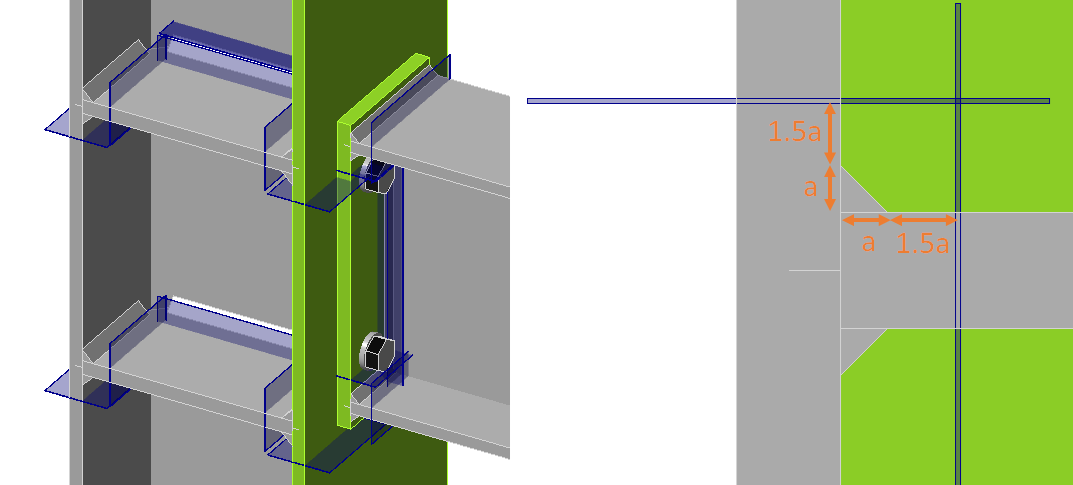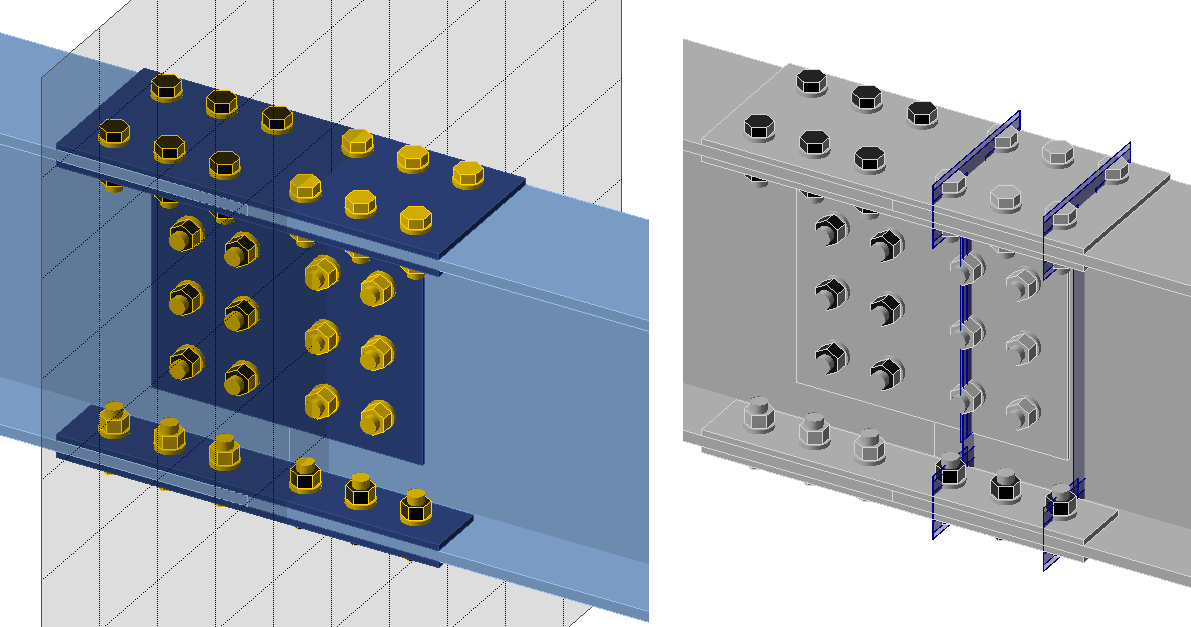# Fatigue analysis type

Tento článek je dostupný také v dalších jazycích:
Fatigue analysis type serves to determine normal and shear stress range between two load cases. The stresses correspond to nominal stresses and must be further evaluated using code design methods. It is assumed to be used for the design of high-cycle fatigue details, where no yielding is expected.
$$The fatigue analysis type does not provide any final resistance or number of cycles the detail can take. It just provides inputs to further calculations according to codes.Always, at least two load cases must be set. The first load case is the reference one. It is assumed as, e.g., a self-weight of the structure and can contain zero loads. The other load cases are simulating fatigue actions. The nominal normal and shear stress provided by IDEA StatiCa is the stress range between the fatigue action, e.g. LE2, and the reference load case.For example, the shear stress at a certain location is 50 MPa in the Reference load case and 180 MPa in LE2. The shown nominal shear stress at this location is:$\tau = 180-50=130\, \textrm{MPa}$Note that there should be no yielding of plates due to fatigue actions, otherwise the stress ranges are distorted. The stresses are available for: Bolts Welds Plates BoltsAt bolts, the stresses are determined simply by dividing the force by the corresponding area: $$\sigma = F_t / A_s$$ $$\tau = V / A$$ where: $$F_t$$ – tensile force in the bolt $$A_s$$ – tensile stress area of the bolt $$V$$ – shear force in bolt; if there are multiple shear planes, the highest shear force is used $$A$$ – area of the bolt resisting in shear; tensile stress area if threads are intercepted by the shear plane and gross cross-sectional area otherwise WeldsWelds in CBFEM consist of the weld element with multipoint constraints connecting the plates. The stress distribution in the weld is disrupted by the constraints and, therefore, the stresses are taken from a section located at 1.5 times the leg size from the weld toe. Three sections are created for a double-sided fillet weld. Two sections are in the same category of detail and only the more stressed one is shown. The maximum normal stress and the corresponding shear stress at the same location, as well as the maximum shear stress and the corresponding normal stress at the same location are shown.PlatesThe stress in plates may be visualized by creating a user-defined section by a Workplane manufacturing operation. In the figure below, two workplanes were created to see the stresses around bolt holes. The maximum normal stress and the corresponding shear stress at the same location, as well as the maximum shear stress and the corresponding normal stress at the same location are shown.$$

## Vyzkoušejte program IDEA StatiCa

Stáhněte si zdarma zkušební verzi na 14 dní.